• 【机器学习－西瓜书】三、线性回归对数线性回归
万次阅读
2017-08-24 10:38:11

墙裂推荐阅读：ｙ的衍生物

关键词：最小二乘法；正则化；对数线性回归； y的衍生物

## 3.1 基本形式

假设样本x有d个属性，线性模型（linear model）试图学得一个通过属性的线性组合来进行预测的函数，即 f(x)=w1x1+w2x2++wdxd+b f ( x ) = w 1 x 1 + w 2 x 2 + ⋅ ⋯ + w d x d + b $f(x)=w_{1}x_{1} + w_{2}x_{2}+\cdot \cdots +w_{d}x_{d}+b$ ，向量形式 f(x)=wTx+b f ( x ) = w T x + b $f(x)=w^{T}x+b$

## 3.2 线性回归

关键词：无序属性连续化。
对离散属性，若属性值之间存在“序”（order）关系，可通过连续化将其转化为连续值，例如二值属性身高的取值，“高”“矮”可和转化为｛1.0 ， 0｝。 若属性值之间不存在序的关系，例如属性“瓜类”的取值为西瓜，南瓜，冬瓜，则可转化为（0，0，1），（0，1，0），（1，0，0）。

关键词：最小二乘法（least square method）。
基于均方误差最小化来进行模型求解的方法称为“最小二乘法”。在线性回归中，最小二乘法就是试图找到一条直线，使所有样本到直线上的欧氏距离之和最小。

关键词： 正则化（regularization）项。
假设解一个线性方程组，当方程数大于自由变量数时，是没有解的。反过来，当方程数小于自由变量数的时候，解就有很多个了。往往，我们会碰到这种情况，参数多，“方程”少的情况，那么有很多个w（权值向量）都能使均方误差最小，那么该选哪一个呢？ 这就涉及到 归纳偏好问题了，常见的做法是引入正则化项。

关键词：对数线性回归（log-linear regression）；y的衍生物
把线性回归模型简写为： f(x)=wTx+b f ( x ) = w T x + b $f(x)=w^{T}x+b$ ，当我们希望线性模型的预测值逼近真实标记y，这样就是线性模型。那可否令模型的预测值毕竟y的衍生物呢？ 作者的这一描述实在太妙了！y的衍生物，通俗易懂！ 假设y的衍生物是 y的对数即lny，那么就可以得到对数线性回归模型： lny=wTx+b l n y = w T x + b $lny=w^{T}x+b$ ， 也就是让模型 去逼近 lny，而不是y。也可以对 lny=wTx+b l n y = w T x + b $lny=w^{T}x+b$ 做一下变换就变成了 y=ewTx+b y = e w T x + b $y=e^{w^{T}x+b}$，也可以理解为让 ewTx+b e w T x + b $e^{w^{T}x+b}$ 去逼近y。形式上还是线性回归的，但实质上已是在求取输入空间到输出空间的非线性函数映射。如图：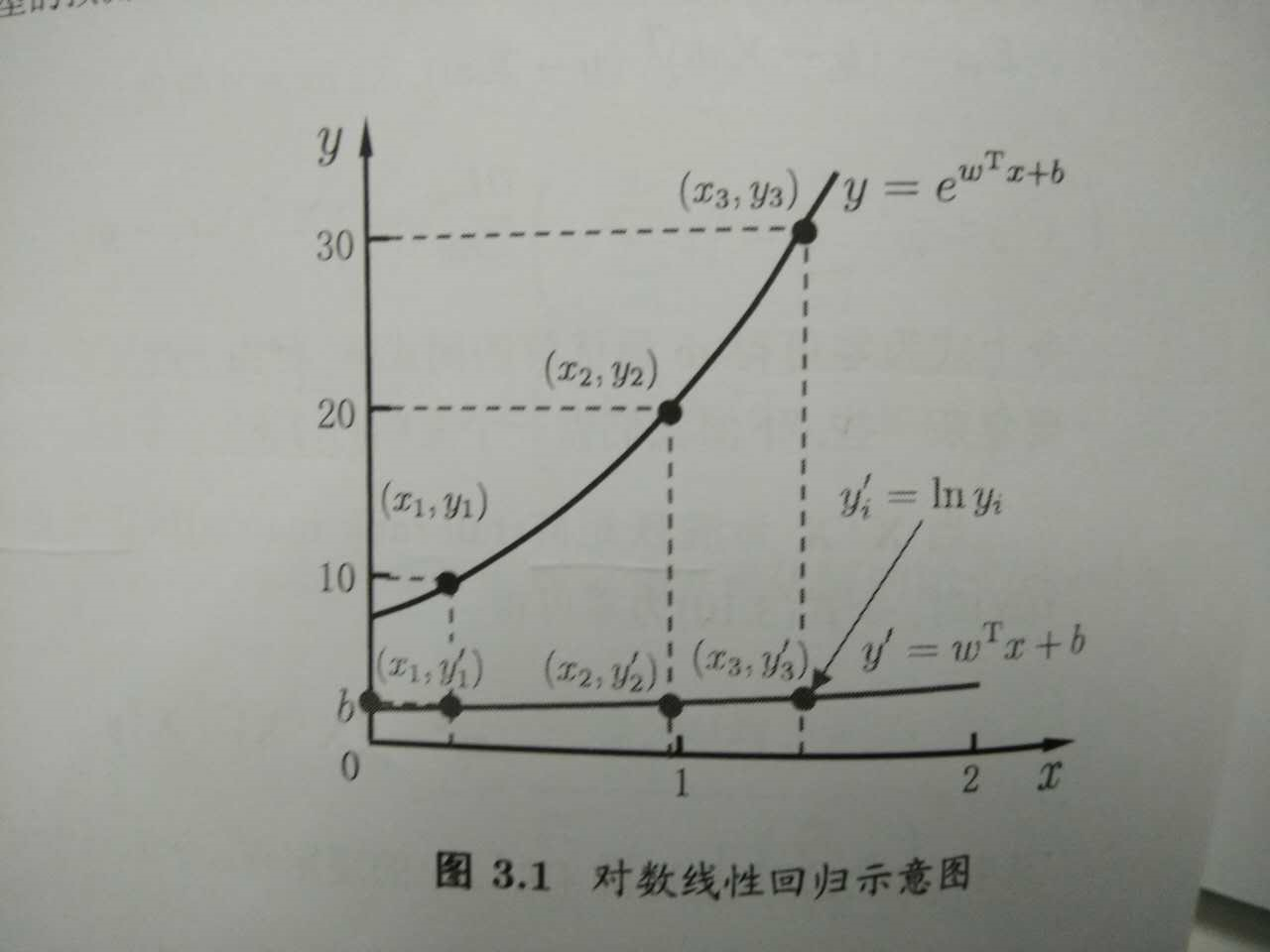来思考一个问题
想从线性模型出发，去扩展线性模型，就是让线性模型 f(x)=wTx+b f ( x ) = w T x + b $f(x)=w^{T}x+b$去拟合y的衍生物，那么我们常说的逻辑回归（对数几率回归）是怎么从线性模型演变而来的呢？是让 wTx+b w T x + b $w^{T}x+b$去拟合哪一种“ｙ的衍生物” 什么呢？这个可以思考思考后，请看下篇：逻辑回归机器学习
更多相关内容
• 公式推导对数几率回归用于处理二分类问题，其数学基础为对数几率函数，是一种 Sigmoid 函数$y = \frac{1}{1+e^{-z}} \tag 1$其函数图像如下取 $z = \boldsymbol{w}^T\boldsymbol{x}+b$，并对式 $(1)$ 进行一定变换...

公式推导

对数几率回归用于处理二分类问题，其数学基础为对数几率函数，是一种 Sigmoid 函数

$y = \frac{1}{1+e^{-z}} \tag 1$

其函数图像如下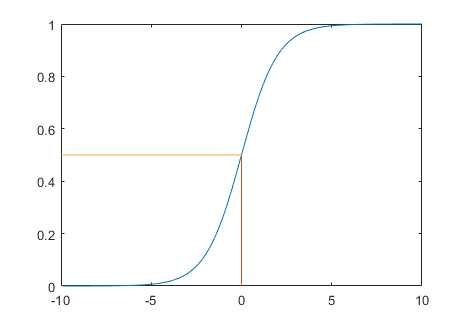取 $z = \boldsymbol{w}^T\boldsymbol{x}+b$，并对式 $(1)$ 进行一定变换，得

$$\ln\frac{y}{1-y}= \boldsymbol{w}^T\boldsymbol{x}+b \tag 2\$$

可以理解为，使用线性回归的预测结果逼近真实标记的对数几率. 当 $y>0.5$ 时，左式大于 $0$；当 $y<0.5$ 时，左式小于$0$.

按照线性回归模型的求解过程，推导出求解 $$\boldsymbol w$$ 和 $$b$$ 的迭代公式. 将式 $$(2)$$ 中的 $$y$$ 看作类后验概率估计$$p(y=1|\boldsymbol x)$$, 代入式 $$(2)$$ 可以推出

\begin{align} p(y=1|\boldsymbol x) = \frac{e^{\boldsymbol w^T\boldsymbol x+b}}{1+e^{\boldsymbol w^T \boldsymbol x+b}} \tag 3\p(y=0|\boldsymbol x) = \frac{1}{1+e^{\boldsymbol w^T \boldsymbol x+b}} \tag 4\\end{align}

令 $$\hat{\boldsymbol w} = (\boldsymbol w;b), \hat{\boldsymbol x_i}=(\boldsymbol x_i;1)$$ 采用极大似然估计法估计 $$\hat{\boldsymbol w}$$ ,最大化函数

$L(\hat{\boldsymbol w}) = \sum\limits_{i=1}^m\ln p(y_i|\hat{\boldsymbol x_i},\hat{\boldsymbol w}) \tag 5\$

令$$p_1(\hat{\boldsymbol x_i},\hat{\boldsymbol w}) = p(y=1|\hat{\boldsymbol x_i},\hat{\boldsymbol w}), p_0 = 1-p_1(\hat{\boldsymbol x_i},\hat{\boldsymbol w})$$，代入式 $$(5)$$，得

$L(\hat{\boldsymbol w}) = \sum\limits_{i=1}^m\ln (y_ip_1(\hat{\boldsymbol x_i},\hat{\boldsymbol w})+(1-y_i)p_0(\hat{\boldsymbol x_i},\hat{\boldsymbol w})) \tag 6\$

代入式 $$(3)(4)$$，最大化式 $$(6)$$ 等价于最小化

$L(\hat{\boldsymbol w}) = \sum\limits_{i=1}^m (-y_i\hat{\boldsymbol w}^T\hat{\boldsymbol x_i}+\ln(1+e^{\hat{\boldsymbol w}^T\hat{\boldsymbol x_i}}))\tag 7\$

使用牛顿迭代法得到迭代更新公式

\begin{align} \hat{\boldsymbol w} &\leftarrow \hat{\boldsymbol w}-\left(\frac{\partial^2L(\hat{\boldsymbol w})}{\partial \hat{\boldsymbol w} \partial\hat{\boldsymbol w}^T} \right )^{-1}\frac{\partial L(\hat{\boldsymbol w})}{\partial\hat{\boldsymbol w}} \tag 8\\frac{\partial L(\hat{\boldsymbol w})}{\partial\hat{\boldsymbol w}} &=-\sum\limits_{i=1}^m \hat{\boldsymbol x_i}(y_i-p_1(\hat{\boldsymbol x_i},\hat{\boldsymbol w})) \tag 9\\frac{\partial^2L(\hat{\boldsymbol w})}{\partial \hat{\boldsymbol w} \partial\hat{\boldsymbol w}^T} &=\sum\limits_{i=1}^m\hat{\boldsymbol x_i}\hat{\boldsymbol x_i}^Tp_1(\hat{\boldsymbol x_i},\hat{\boldsymbol w})(1-p_1(\hat{\boldsymbol x_i},\hat{\boldsymbol w})) \tag {10} \end{align}

其中式 $$(9)$$ 可以向量化为

$\frac{\partial L(\hat{\boldsymbol w})}{\partial\hat{\boldsymbol w}} = \boldsymbol X^T(p_1({\boldsymbol X},\hat{\boldsymbol w})-\boldsymbol y) \tag{11}$

MATLAB 实现

% 生成随机训练样本，直线 y=0.7x+200 上方的为正例，下方为反例

% 输出训练样本在坐标轴上的分布

x = zeros(100, 2);

y = zeros(100, 1);

kb = [0.7,200];

figure;

hold on;

for i = 1:100

x(i,1) = randi(1000,1);

x(i,2) = randi(1000,1);

if kb(1)*x(i,1)+kb(2)>x(i,2)

plot(x(i,1), x(i,2), ‘r*‘);

y(i) = 1;

else

plot(x(i,1),x(i,2), ‘b*‘);

y(i) = 0;

end

end

% 牛顿迭代法解权重

function w = cal(X,y,eps)

[m,n] = size(X);

X = [X ones(m,1)];

n = n + 1;

w = zeros(n, 1);

w(3) = 1000;

prew = zeros(n, 1);

while (true)

flag = 0;

sum1 = X‘*(1-1./(1+exp(X*prew))-y);

sum2 = 0;

for i = 1:m

sum2 = sum2 + X(i,:)*X(i,:)‘*(1-1./(1+exp(X(i,:)*prew)))*(1./(1+exp(X(i,:)*prew)));

end

w = prew - sum1./sum2;

for i = 1:n

if abs(w(i)-prew(i))>eps

flag = 1;

end

end

if flag==0

break;

end

prew = w;

end

end

% 测试并输出训练效果图像

eps = 0.0001;

w = cal(x, y, eps);

figure;

hold on;

for i=1:100

yy = w(1)*x(i,1)+w(2)*x(i,2)+w(3);

if yy>0

plot(x(i,1), x(i,2), ‘r*‘);

else

plot(x(i,1), x(i,2), ‘b*‘);

end

end

训练集的真实分布(红色正例、蓝色反例)：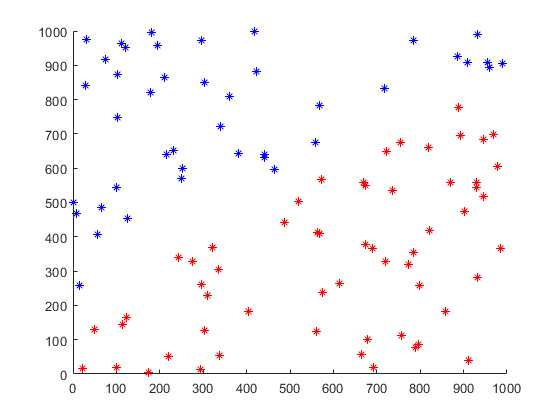模型的分类效果：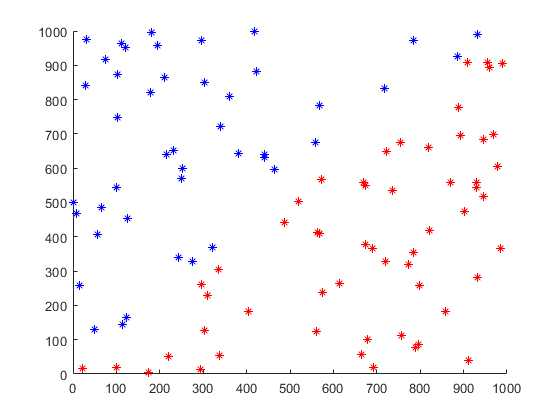原文：https://www.cnblogs.com/Kusunoki/p/13817347.html

展开全文• sklearn:线性回归与逻辑回归对数几率回归

在本次学习中,以实践为主,简要阐述理论原理即可

# 1. 线性回归(LinearRegression)

## 1.1 理论

给定数据集D = { ( x 1 , y 1 ) , ( x 2 . y 2 ) , . . . , ( x m , y m ) (x_1, y_1), (x_2. y_2), ... , (x_m, y_m) }, 其中 x i = ( x i 1 . x i 2 , . . . , x i d ) , y i ∈ R x_i = (x_{i1}. x_{i2}, ..., x_{id}), y_i \in R

1. 目的:试图学得一个线性模型以尽可能准确的预测实值输出标记
2. 模型的基本形式: f ( x ) = w x i + b f(x) = wx_i + b
3. 常用指标:均方误差(基于均方误差最小化来进行模型求解的方法为"最小二乘法")
4. 损失函数:通过使均方误差最小化得到的最小二乘损失函数
5. 求解:通过梯度下降法求解出使损失函数最小时所对应的参数
6. 梯度下降法的一般过程: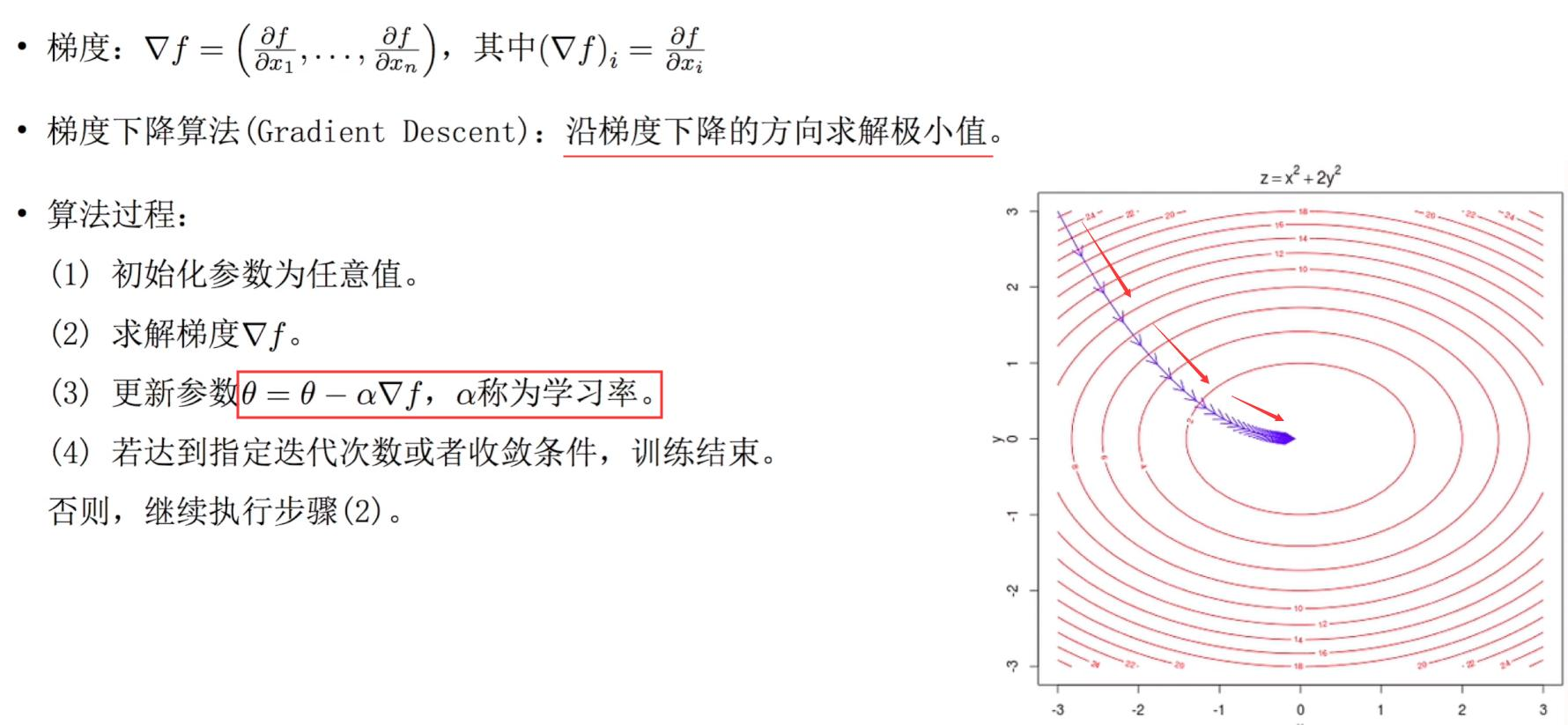## 1.2 重要参数

参数意义
fit_intercept布尔值，可不填，默认为True 是否计算此模型的截距。如果设置为False，则不会计算截距
normalize布尔值，可不填，默认为False。当fit_intercept设置为False时，将忽略此参数。如果为True，则特征矩阵X在进入回归之前将会被减去均值（中心化）并除以L2范式（缩放）
copy_X布尔值，可不填，默认为True。如果为真，将在X.copy()上进行操作，否则的话原本的特征矩阵X可能被线性回归影响并覆盖
n_jobs用于计算的作业数

## 1.3 实践

以波士顿房价为例

1. 导入相关的包
# 1. 导入相关的包
import numpy as np
import pandas as pd

1. 导入数据集
# 2. 导入波士顿房价数据集
# 3. 加载相关数据
X = data.data
y = data.target

1. 划分训练集和测试集
# 3. 划分训练集和测试集
from sklearn.model_selection import train_test_split
X_train, X_test, y_train, y_test = train_test_split(X_data, y_data, test_size= 0.3, random_state = 0)
print(X_train.shape, X_test.shape,y_train.shape, y_test.shape)

1. 导入模型并训练
# 4. 导入相关模型并训练
from sklearn.linear_model import LinearRegression
# 实例化
lr = LinearRegression()
# 训练
lr.fit(X_train, y_train)

# 输出对应线性回归的系数

print('线性回归的系数为:\n w = %s \n b = %s' % (lr.coef_, lr.intercept_))

1. 利用学习好的模型进行预测
# 5. 利用学的模型进行预测
y_test_pred = lr.predict(X_test)

1. 画出预测与真实值的图像
plt.figure()
plt.plot(y_test, c = 'r')
plt.plot(y_test_pred, c = 'b')
plt.show()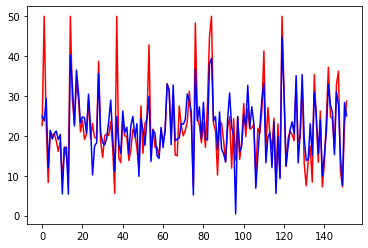1. 计算均方误差
from sklearn.metrics import mean_squared_error
mean_squared_error( y_test, y_test_pred)


结果为27.2

# 2. 对数几率回归(LogisticRegression,也被称为逻辑回归)

给定数据X = x 1 , x 2 , . . . , Y = y 1 , y 2 , . . . x_1, x_2, ... , Y = y_1, y_2, ... ,考虑为二分类任务，即 y i ∈ 0 , 1 y_i \in 0, 1

## 2.1 理论

1. 定义:是一种名为“回归”的线性分类器，其本质是由线性回
归变化而来的，一种广泛使用于分类问题中的广义回归算法。
2. 目的：通过对线性模型进行Sigmoid变换完成分类任务
3. 基本形式： h θ ( x ) = g ( θ T x ) h_{\theta}(x) = g(\theta^Tx) , 其中 θ T = w T x + b , g ( z ) = 1 1 + e − z \theta ^T = w^Tx + b, g(z) = \frac{1}{1 + e^{-z}}
4. 损失函数：通过使用极大似然估计法定义损失函数
5. 参数的求解：根据凸优化理论，可以使用梯度下降法得出最优解

## 2.2 LogisticRegression的优点：

1. 对线性关系的拟合效果极好（特征与标签之前的线性关系极强的数据，比如金融领域中的信用卡欺诈、评分卡制作以及营销预测等）
2. 计算速度快
3. 返回的分类结果不是固定的0和1，而是以小数呈现出来的类概率数组

## 2.3 重要参数及其意义

参数意义
penalty可以通过输入’l1’或’l2’来指定使用哪一种正则化方式，默认为’l2’
C正则化强度的倒数，必须是一个大于0的浮点数，不填写默认为1.0.越小，损失函数会越小。模型对损失函数的惩罚越重，正则化的效力越强
max_iter整数，默认为100，求解器收敛的最大迭代次数
multi_class输入"ovr"（二分类）, “multinomial”（多分类）, “auto”（根据数据的分类情况和其他参数来确定模型要处理的分类问题的类型）来告知模型处理的分类问题的类型，默认是"ovr"
solver求解参数的方法选择，
class_weight针对样本不均衡问题的参数，不过使用较难，一般采用上采样或下采样来解决样本不均衡问题

## 2.4 实践

以乳腺癌数据为例

1. 导入相关的包
# 1. 导入相关的包
import numpy as np
import pandas as pd

1. 导入数据集
from sklearn.datasets import load_breast_cancer
X = data.data
y = data.target

1. 划分训练集和测试集
from sklearn.model_selection import train_test_split
X_train, X_test, y_train, y_test = train_test_split(X, y, test_size=0.2, random_state = 0)

1. 导入模型并训练
from sklearn.linear_model import LogisticRegression
lgr = LogisticRegression()
lgr.fit(X_train, y_train)

1. 预测并查看得分
y_pred = lgr.predict(X_test)
score = lgr.score(X_test, y_test)
score


结果为0.947

展开全文sklearn 机器学习
• 首先我们来看为什么Logistic Regression被称为对数几率回归。 几率：将一个实例映射到正例或者负例的可能性比率。令P(y=1|x;θ)P(y=1|x;θ)P(y = 1 | x; \theta)表示将样本分类成正例的概率。那么P(y=0|x...

Logistic Regression是一个经典的判别学习分类方法。参考资料—西瓜书&&机器学习公开课-Andrew Ng。
首先我们来看为什么Logistic Regression被称为对数几率回归。

几率：将一个实例映射到正例或者负例的可能性比率。令 P(y=1|x;θ) P ( y = 1 | x ; θ ) $P(y = 1 | x; \theta)$表示将样本分类成正例的概率。那么 P(y=0|x;θ)=1P(y=1|x;θ) P ( y = 0 | x ; θ ) = 1 − P ( y = 1 | x ; θ ) $P(y = 0 | x; \theta) = 1 - P(y = 1 | x; \theta)$就表示将样本分类成负例的概率。那么这里的几率表示为：
P(y=1|x;θ)1P(y=1|x;θ) P ( y = 1 | x ; θ ) 1 − P ( y = 1 | x ; θ ) $\frac{P(y = 1 | x; \theta)}{1 - P(y = 1 | x; \theta)}$

对数几率：对数几率就是对上式求对数。

回归就是线性回归方法。因此：对数几率回归的表达式如下：
logP(y=1|x;θ)1P(y=1|x;θ)=θTx l o g P ( y = 1 | x ; θ ) 1 − P ( y = 1 | x ; θ ) = θ T x $log \frac{P(y = 1 | x; \theta)}{1 - P(y = 1 | x; \theta)} = \theta^Tx$
经过简单的代数运算，我们得到
P(y=1|x;θ)=eθTx1+eθTx P ( y = 1 | x ; θ ) = e θ T x 1 + e θ T x $P(y = 1 | x; \theta) = \frac{e^{\theta^Tx}}{1 + e^{\theta^Tx}}$；以及
P(y=0|x;θ)=11+eθTx P ( y = 0 | x ; θ ) = 1 1 + e θ T x $P(y = 0 | x; \theta) = \frac{1}{1 + e^{\theta^Tx}}$

我们看到 P(y=1|x;θ) P ( y = 1 | x ; θ ) $P(y = 1 | x; \theta)$ P(y=0|x;θ) P ( y = 0 | x ; θ ) $P(y = 0 | x; \theta)$的表达式实际上就是Sigmoid函数。那么为什么要这样做呢？

通过线性回归的方法做分类，理想的情况下是这样来做：

z=θTx z = θ T x $z = \theta^Tx$，当z<0，样本被分类成负例；当z>0，样本被分类成正例；当z=0，样本可以任意分类。显然，这里预测函数为单位阶跃函数

y=hθ(x)=g(z)=0.1,z>0,5,z=0,0,z<0(33) (33) y = h θ ( x ) = g ( z ) = { 1 , z > 0 , 0. 5 , z = 0 , 0 , z < 0

g(z)的函数图像如下图红线所示：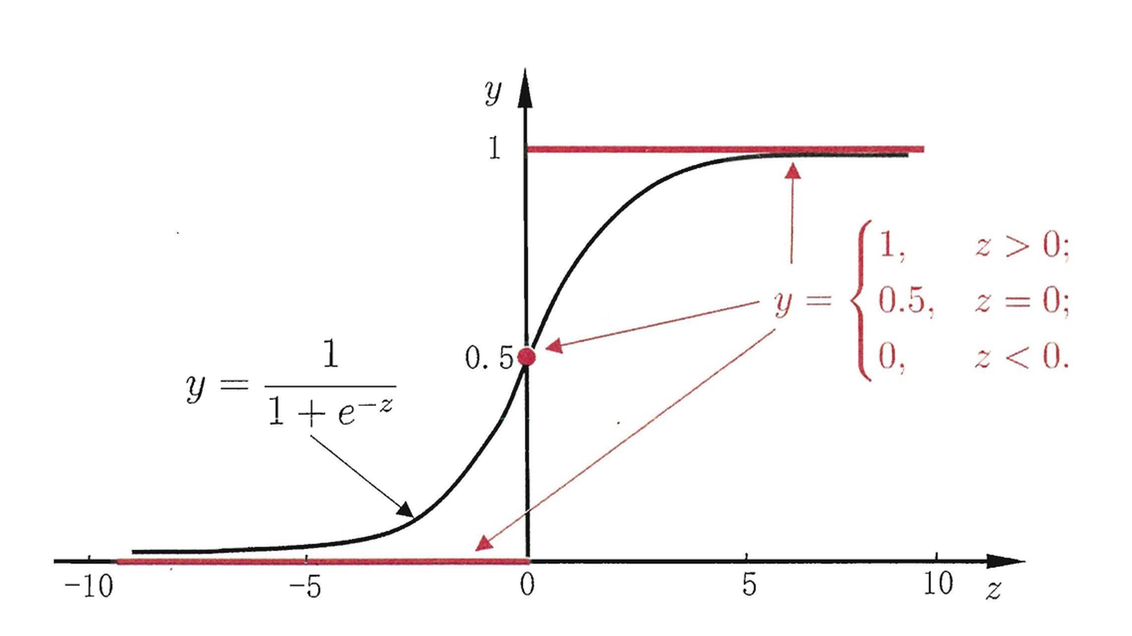而单位阶跃函数是不连续不可微的，这对于我们求解参数带来了很大的不便。
因此，科学家们就想到用另外一个连续可微函数近似替代这个阶跃函数。常用的替代函数如上图黑线所示，它也被称为Sigmoid函数，也可以被称为对数几率函数。同样地，当对数几率函数值大于0.5，样本被分类成正例；小于0.5，样本被分类成负例；等于0.5，样本可被任意分类。

上面就是对对数几率函数的一些解释。咱们继续，在得到了 P(y=1|x;θ) P ( y = 1 | x ; θ ) $P(y = 1 | x; \theta)$ P(y=0|x;θ) P ( y = 0 | x ; θ ) $P(y = 0 | x; \theta)$之后，我们得到后验概率如下，对某一个训练样本：

P(yi|xi;θ)=yiP(yi=1|xi;θ)+(1yi)P(yi=0|xi;θ) P ( y i | x i ; θ ) = y i P ( y i = 1 | x i ; θ ) + ( 1 − y i ) P ( y i = 0 | x i ; θ ) $P(y_i | x_i; \theta) = y_i P(y_i = 1 | x_i; \theta) + (1 - y_i) P(y_i = 0 | x_i; \theta)$

写出对数似然函数：
L(θ)=logni=1P(yi|xi;θ)=ni=1logP(yi|xi;θ) L ( θ ) = l o g ∏ i = 1 n P ( y i | x i ; θ ) = ∑ i = 1 n l o g P ( y i | x i ; θ ) $L(\theta) = log\prod_{i = 1}^n P(y_i | x_i; \theta) = \sum_{i = 1}^n logP(y_i | x_i; \theta)$

诶 忽然发现有些不对，
logP(yi|xi;θ)=log[yiP(yi=1|xi;θ)+(1yi)P(yi=0|xi;θ)] l o g P ( y i | x i ; θ ) = l o g [ y i P ( y i = 1 | x i ; θ ) + ( 1 − y i ) P ( y i = 0 | x i ; θ ) ] $log P(y_i | x_i; \theta) = log[y_i P(y_i = 1 | x_i; \theta) + (1 - y_i) P(y_i = 0 | x_i; \theta)]$不好处理。于是，我们改写:
P(yi|xi;θ) P ( y i | x i ; θ ) $P(y_i | x_i; \theta)$
=yiP(yi=1|xi;θ)+(1yi)P(yi=0|xi;θ) = y i P ( y i = 1 | x i ; θ ) + ( 1 − y i ) P ( y i = 0 | x i ; θ ) $= y_i P(y_i = 1 | x_i; \theta) + (1 - y_i) P(y_i = 0 | x_i; \theta)$
=P(yi=1|xi;θ)yiP(yi=0|xi;θ)1yi = P ( y i = 1 | x i ; θ ) y i P ( y i = 0 | x i ; θ ) 1 − y i $= P(y_i = 1 | x_i; \theta)^{y_i}P(y_i = 0 | x_i; \theta)^{1-y_i}$
于是,
L(θ)=ni=1[yilogP(yi=1|xi;θ)+(1yi)logP(yi=0|xi;θ)] L ( θ ) = ∑ i = 1 n [ y i l o g P ( y i = 1 | x i ; θ ) + ( 1 − y i ) l o g P ( y i = 0 | x i ; θ ) ] $L(\theta) = \sum_{i = 1}^n [y_i logP(y_i = 1 | x_i; \theta) + (1 - y_i)logP(y_i = 0 | x_i; \theta)]$
=ni=1[yilogeθTxi1+eθTxi+(1yi)log11+eθTxi] = ∑ i = 1 n [ y i l o g e θ T x i 1 + e θ T x i + ( 1 − y i ) l o g 1 1 + e θ T x i ] $=\sum_{i = 1}^n [y_i log\frac{e^{\theta^Tx_i}}{1 + e^{\theta^Tx_i}} + (1 - y_i)log\frac{1}{1 + e^{\theta^Tx_i}}]$
=ni=1[yi(θTxilog(1+eθTxi))(1yi)log(1+eθTxi)] = ∑ i = 1 n [ y i ( θ T x i − l o g ( 1 + e θ T x i ) ) − ( 1 − y i ) l o g ( 1 + e θ T x i ) ] $=\sum_{i = 1}^n[y_i(\theta^Tx_i - log(1 + e^{\theta^Tx_i})) - (1 - y_i)log(1 + e^{\theta^Tx_i})]$
=ni=1[yiθTxilog(1+eθTxi)] = ∑ i = 1 n [ y i θ T x i − l o g ( 1 + e θ T x i ) ] $=\sum_{i = 1}^n[y_i\theta^Tx_i - log(1 + e^{\theta^Tx_i})]$
极大化对数似然等价于极小化其负数

minθL(θ)=ni=1[yiθTxi+log(1+eθTxi)]=L̂ (θ) m i n θ − L ( θ ) = ∑ i = 1 n [ − y i θ T x i + l o g ( 1 + e θ T x i ) ] = L ^ ( θ ) $min_\theta -L(\theta) = \sum_{i = 1}^n[-y_i\theta^Tx_i + log(1 + e^{\theta^Tx_i})] = \hat{L}(\theta)$

从损失函数的角度也可以导出上述式子

定义损失函数

Cost(hθ(x),y)={log(hθ(x)),y=1,log(1hθ(x)),y=0.(34) (34) C o s t ( h θ ( x ) , y ) = { − l o g ( h θ ( x ) ) , y = 1 , − l o g ( 1 − h θ ( x ) ) , y = 0.

化简得到 Cost(hθ(x),y)=log[(hθ(x))y(1hθ(x))1y] C o s t ( h θ ( x ) , y ) = − l o g [ ( h θ ( x ) ) y ( 1 − h θ ( x ) ) 1 − y ] $Cost(h_\theta(x), y) = -log[(h_\theta(x))^y(1 - h_\theta(x))^{1 - y}]$
训练数据总体损失
J(θ)=ni=1Cost(hθ(xi),yi)=ni=1[yiloghθ(xi)+(1yi)log(1hθ(xi))]=L̂ (θ) J ( θ ) = ∑ i = 1 n C o s t ( h θ ( x i ) , y i ) = − ∑ i = 1 n [ y i l o g h θ ( x i ) + ( 1 − y i ) l o g ( 1 − h θ ( x i ) ) ] = L ^ ( θ ) $J(\theta) = \sum_{i = 1}^n Cost(h_\theta(x_i), y_i) = -\sum_{i = 1}^n [ y_ilogh_\theta(x_i) + (1 - y_i)log(1 - h_\theta(x_i))] = \hat{L}(\theta)$

最终目标为
minθJ(θ) m i n θ J ( θ ) $min_\theta J(\theta)$
目标函数对 θ θ $\theta$求偏导可得
θJ(θ)=ni=1[yixi+xieθTxi1+eθTxi] ∂ ∂ θ J ( θ ) = ∑ i = 1 n [ − y i x i + x i e θ T x i 1 + e θ T x i ] $\frac{\partial}{\partial\theta}J(\theta) = \sum_{i = 1}^n [-y_ix_i + \frac{x_ie^{\theta^Tx_i}}{1 + e^{\theta^Tx_i}}]$
这个式子的解析解不容易给出，于是利用标准梯度下降法给出 θ θ $\theta$的迭代公式如下：

θ(j+1):=θ(j)αθJ(θ(j)) θ ( j + 1 ) := θ ( j ) − α ∂ ∂ θ J ( θ ( j ) ) $\theta^{(j+1)} := \theta^{(j)} -\alpha \frac{\partial}{\partial\theta}J(\theta^{(j)})$

当然，也可以通过牛顿法，随机梯度下降等其他经典的方法求解。

下面附上代码（Python实现），建议在PyCharm下运行，数据集采用的是《机器学习实战》的Logistic回归测试数据：

# -*- coding:utf-8 -*-
# This class is built for logistic regression
__author__ = 'yanxue'

import numpy as np
from numpy import random
from numpy import linalg
from matplotlib import pyplot as plt
import math

class LogisticRegression:
def __init__(self):
pass

def learning(self, data, label, learning_rate=0.1, convergence_rate=1):
"""Learning process of the logistic regression"""
n, d = data.shape
self.theta = random.randn(d + 1)
theta0 = random.randn(d + 1)
count = 0
c_error = 0
for j, x in enumerate(data):
pLabel = lr.classify(x)
c_error += pLabel != label[j]
yield c_error, theta0
ind = 0
while linalg.norm(theta0 - self.theta) > convergence_rate:
print('theta_{} = {}'.format(count, theta0))
self.theta = theta0.copy()
theta0 -= learning_rate * sum(map(self.__fun, [(data[i], label[i]) for i in range(n)]))
# theta0 -= learning_rate * self.__fun((data[ind % n], label[ind % n]))
# ind += 1
# theta0 -= learning_rate * sum(map(self.__fun, [(data[i % n], label[i % n]) for i in range(ind, ind + 10)]))
# ind += 10
c_error = 0
for j, x in enumerate(data):
pLabel = lr.classify(x)
c_error += pLabel != label[j]
yield c_error, self.theta
count += 1

def __fun(self, trainx):
return -trainx * np.append(trainx, 1) + np.append(trainx, 1) * self.s(trainx)

def classify(self, x):
if self.s(x) < 0.5:
return 0
elif self.s(x) >= 0.5:
return 1

def s(self, inx):
"""Sigmoid function value"""
try:
re = 1 / (1 + math.exp(-np.dot(self.theta, np.append(inx, 1))))
except OverflowError as e:
print(e)
re = 1
return re

def __str__(self):
return "Logistic regression"

trainX = trainData[:, [0, 1]]
trainY = trainData[:, 2]
lr = LogisticRegression()
# ------ Plot the data points ------
x1 = np.arange(-4.0, 3.2, 0.1)
plt.close()
plt.ion()  #interactive mode on
fig = plt.figure()
# ------ End of plot ------
for c_error, c_theta in lr.learning(trainX, trainY):
# ------ Plot the iterative process ------
plt.grid()
plt.scatter(trainX[trainY == 0, 0], trainX[trainY == 0, 1], c='r')  # plot positive instances
plt.scatter(trainX[trainY == 1, 0], trainX[trainY == 1, 1], c='b')  # plot negative instances
plt.xlabel('$x_1$')
plt.ylabel('$x_2$')
plt.title('Logistic Regression Demo')
x2 = (-c_theta * x1 - c_theta) / c_theta
plt.plot(x1, x2)
plt.text(-4, 12.5, 'errorNum = {}'.format(c_error))
# ------ End of plot ------
plt.pause(0.2)
展开全文Logistic
• 1 对数几率回归原理分析 1.1 引入 1.2 损失函数 1.3 求最优解 2 对数几率回归实践 Logistic回归的一般过程 Logistic回归的优缺点 Logistic回归算法描述（改进的随机梯度下降） 《机器学习实战》第五章代码...机器学习 机器学习实战 分类
• 《机器学习：公式推导与代码实践》鲁伟著读书笔记。...在对数几率回归中，我们需要将线性回归模型的预测值转化为0/1值，而不是去逼近真实标签yyy。而取值范围为（0，1），单调可微的Sigmoid函数便是对机器学习 python 分类算法
• 使用方法： 首先输入实验数据的对数 （一个x和一个y算一对） 然后输入x值和y值 全部输入结束时会被询问是否修改实验数据。如果修改，输入y，否则输入n 然后输入B类不确定度，随后显示最终结果exe格式
• 对数几率回归（logistic regression），有时候会译为逻辑回归(音译)，其实是我们把回归模型应用到分类问题时，线性回归的一种变形，主要是针对二分类提出的。既然是线性回归的一种变形，那么在理解对数几率回归时，...
• 对数几率回归 又常常称为逻辑回归，逻辑斯谛回归 如果是分类任务，如何使用线性回归模型呢？答案在广义线性模型的公式中，只需要找到一个单调可微函数将分类任务的真实标记y 与线性回归模型的预测值联系起来。 考虑...
• 它是广义的线性模型，只是将线性回归方程中的y换成了ln[p/(1-p)，p是p(y=1|x)，p/(1-p)是“几率”。对数几率回归是用来做分类任务的，所以，需要找一个单调可微函数，将分类任务的真实标记和线性回归模型的预测值...机器学习
• 通常求解线性回归方程，在Excel中使用的方法为规划求解，今天我从另外两个思路给你讲一讲如何求解线性回归方式给。先来看一下什么是线性回归方程：线性回归方程是利用数理统计中的回归分析，来确定两种或两种以上...
• 书接上回，我们可以对一个数据集得到它的回归方程 我们是这样去解读回归方程的：在相关属性下对样例的某一特征的度量 我们根据回归方程得到的特征度量是一个连续值，我们可以根据这个度量值进行分类 例如：大学的...机器学习 梯度下降法
• 线性模型即用线性代数中的线性方程表示一个模型，诸如fx=wTx+b的形式。 其中训练得出w和b后，模型就得以确认。 其中w其实就是代表各个属性的权重值。 线性模型有三种任务，分别为回归任务、二分类任务和多分类任务。...机器学习
• 对数几率回归模型是处理分类问题的算法，常用于垃圾邮件分类，天气预测等，很多文献也将其称为“逻辑回归”。 本文也将称为逻辑回归。 一. 逻辑回归由来 下面是一个简单的线性回归模型。   我们知道“线性回归...
• 对数几率回归原理代码实现 原理 之前介绍了 代码实现机器学习
• 回归方程是通过分析样本数据得到的变量间的回归关系的数字表达式。回归方程拟合程度足够好的话，可运用自变量来预测因变量的数值。比如，我们经常会构建销售额与客流量间的回归方程，以预测一定客流量下的销售额。 ...
• ## 对数几率回归 —— Logistic Regression

万次阅读 多人点赞 2018-07-02 16:13:12
机器学习基础算法python代码实现可参考：zlxy9892/ml_code1 原理1.1 引入首先，在引入LR(Logistic Regression)模型之前，非常重要的一个概念是，该模型在设计之初是用来解决0/1二分类问题，虽然它的名字中有回归二字...
• 预测区间估计(prediction interval estimate)：利用估计的回归方程，对于自变量 x 的一个给定值 x0 ，求出因变量 y 的一个个别值的估计区间。变量的估计叫预测区间，预测区间反映了单个数值的不确定性； 置信...置信区间 预测区间
• 对数几率回归(逻辑斯蒂回归) @(机器学习经典算法总结) 逻辑回归基本是我们所有人学习的第一个分类器，分类器从概率意义上理解，代表的是什么意思，以最简单的二分类来举例吧，假设样本数据 D=(x(i),y(i)),&...
• 相信很多同学在学习对数几率回归（逻辑回归）时，都会有很多困惑，比如： 1.对数几率回归（逻辑回归）到底是用来干嘛的？ 2.sigmoid函数是用来干嘛的？ 3.z值为什么表示样本点属于其中一个类别的概率？ 4.预测出的y...机器学习 lr
• 逻辑回归 逻辑回归的模型是一个非线性模型 sigmoid函数，又称逻辑回归函数。但是它本质上又是一个线性回归模型 因为除去sigmoid映射函数关系，其他的步骤，算法都是线性回归 可以说，逻辑回归，都是以线性回归为...python 算法 机器学习 人工智能
• R构建对数回归模型（Logarithmic Regression） 目录 R构建对数回归模型（Logarithmic Regression） 对数回归模型（Logarithmic Regression） 创建数据 数据可视化 拟合对数回归模型 对数回归模型...R 数据挖掘 机器学习 人工智能 深度学习
• 我总是告诉学生，采用自然对数来转换变量的三个原因。记录变量的原因将决定您是要记录独立变量还是因变量或两者。为了清楚起见，我正在谈论采用自然对数。首先，如其他海报所指出的那样，提高模型拟合度。例如，如果...
• 对数几率回归的定义 1.1 广义线性模型 在之前的机器学习(一)：深入解析线性回归模型一文中所介绍的线性模型都是基于y=wTx+by=w^Tx+by=wTx+b这种形式的。但对于某些数据集虽然其自变量xxx与因变量yyy并不满足一元函数...机器学习 python 算法
• 对数几率回归1.对数几率回归 的广义线性模型推导2.极大似然估计函数3.对数几率回归的参数估计 1.广义线性模型 1.指数族分布 2.广义线性模型的三条假设 2.对数几率回归 1.对数几率回归 的广义线性模型推导 2.极大似然...机器学习 算法
• ## 16回归方程的验证

千次阅读 2019-07-30 08:04:56
1.回顾回归方程的意义   首先，我们指明回归方程的通式： y=β0+β1x+εy=\beta_{0}+\beta_{1} x+\varepsilony=β0​+β1​x+ε ①β0\beta_0β0​和β1\beta_1β1​表示模型参数，分别对应截距和斜率。这些值...机器学习与数据挖掘
• BG：在box-cox变换中，当λ = 0时即为对数变换。当所分析变量的标准差相对于均值而言比较大时，这种变换特别有用。对数据作对数变换常常起到降低数据波动性和减少不对称性的作用。。这一变换也能有效消除异方差性...
• 首先，对数几率回归和线性回归的一点直观上的理解： 线性回归目的是找到一条直线（或者超平面）尽可能地接近所有的训练数据点，而对数几率回归（二元）的目的是找到一条直线（或者超平面）尽可能地分开两种不同...
• 1.1 因变量采用对数变换 ln(y^)=β0+β1×xln(\hat y)=\beta_0 +\beta_1 \times xln(y^​)=β0​+β1​×x x→x+1;y^1→y^2x \to x+1; \hat y_1 \to \hat y_2x→x+1;y^​1​→y^​2​ {y^1=eβ0+β1×xy^2=eβ0+β1......